Function Repository Resource:

# CoefficientMap

Map a function over the coefficients of a polynomial

Contributed by: Daniel Robinson and Jon McLoone
 ResourceFunction["CoefficientMap"][f,p] maps the function f over the coefficients of polynomial p. ResourceFunction["CoefficientMap"][f,p,vars] maps the function f over the coefficients of polynomial p in variables vars.

## Examples

### Basic Examples (2)

Map an arbitrary function over the coefficients of a polynomial:

 In:=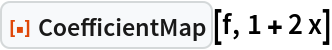Out=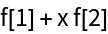Take the square root of the coefficients of a polynomial:

 In:=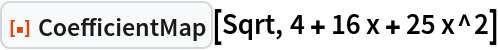Out=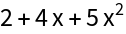### Scope (2)

By default none of the variables identified by Variables will be considered part of the coefficient:

 In:=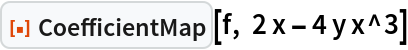Out=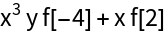When specifying the variables of the polynomial using a third arguments, other symbols will be considered part of the coefficient:

 In:=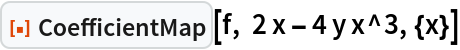Out=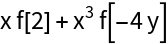Jon McLoone

## Version History

• 1.0.0 – 05 July 2022